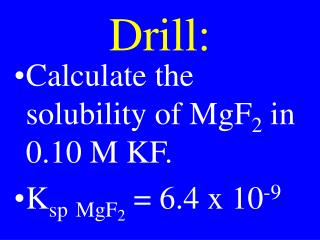DownloadDownload PresentationDrill:

# Drill:

Download Presentation## Drill:

- - - - - - - - - - - - - - - - - - - - - - - - - - - E N D - - - - - - - - - - - - - - - - - - - - - - - - - - -
##### Presentation Transcript

1. Drill: • Calculate the solubility of MgF2 in 0.10 M KF. • KspMgF2 = 6.4 x 10-9

2. Nuclear Chemistry

3. Nuclear Chemistry • The study of reactions that take place in the nuclei of atoms

4. Chemical Reactions • In normal chemical reactions, only the electrons are involved

5. Radioactive Nuclei • A nucleus that spontaneously decomposes

6. Isotopes • Elements with the same atomic number, but different mass number

7. Isotopes • Elements with = numbers of protons, but  numbers of neutrons

8. Isotopes • All elements have at least one radioactive isotope

9. Radiation • The emission of particles & rays from spontaneously decomposing nuclei

10. Modes of Decay • Alpha emission • Beta emission • Gamma emission • Positron emission • K-electron capture

11. Drill: • Name five types of radiation

12. Drill: • Name 3 common types of radiation

13. Alpha Particle (a) • Helium nucleus • 2 protons & 2 neutrons • mass = 4 amu • charge = +2 • Penetration power: small

14. Beta Particle (b) • High speed electron • 1 electron • mass = 1/1836 amu • charge = -1 • Penetration power: medium

15. Gamma Ray (g) • High energy photon • Electromagnetic wave • mass = 0 • charge = 0 • Penetration power: great

16. Positron (p) • Positive electron • 1 positive electron • mass = 1/1836 amu • charge = +1 • Penetration power: medium

17. K-capture • The capture of an inner level e- by the nucleus • 1 electron • mass = 1/1836 amu • charge = -1

18. Nuclear Symbol • Alpha: 24Heor24a • Beta: -10eor –10b • Gamma: 0 0 • Positron: +10e • K-electron: -10e

19. Fission • The splitting of a nucleus into smaller nuclei involving the release of energy

20. Fusion • The combining of smaller nuclei into a larger one involving the release of energy

21. Transmutation Rxns • Nuclear reactions in which one element is changed into another

22. Transmutation Rxns • Reactions in which the nucleus of an atom is changed

23. Transmutation Rxns • Both fission & fusion are examples of transmutation rxns

24. Transmutation Rxns • Can occur through emission of or bombardment by radioactive particles

25. Transmutation Rxns • b emission of Pm-142 • a bombardment of Th-231

26. AP CHM HW • Read: Chapter 19 • Problems: 18 & 19 • Page: 553

27. CHM II HW • Read: Chapter 26 • Problems: 31 & 35 • Page: 1036

28. Transmutation Rxns • a emission ofU-238 followed by two separate b emissions:

29. Transmutation Rxns • a bombardment ofTh-235 followed by two separate b emission:

30. Drill: Predict Prod • Neutron absorption by U-238 followed by two separate b emission:

31. Predict Products • a emission of O-18 followed by a • b emission:

32. Predict Products • K-capture by V-45 followed by neutron emission then a emission

33. Predict Products • b absorption by V-45 followed by neutron emission then a emission

34. Decay Rate • The rate at which radioactive nuclei break down

35. Half-Life • The time it takes for 50 % of the radioactive nuclei to decompose

36. Decay Rate • Rate = kdX/dt • ln(Xo/X) = kt1/2 • k = 0.693/t1/2 • t1/2 = half-life

37. Drill: Predict the products in each step when Boron-12 goes through a bombardment followed by b emission.

38. 1st Order Age Dating Formula • t = ln(Xi/Xf)t1/2 • 0.693

39. Calculate the age of a skeleton found with 0.125 % C-14 when atmospheric C-14 = 1.00 %. • t1/2 C-14 = 5720 yr

40. Calculate the age of a tooth found with 0.00132 % C-14 when atmospheric C-14 = 1.00 %. t1/2 C-14 = 5720

41. AP CHM HW • Read: Chapter 19 • Problems: 21 & 22 • Page: 553

42. CHM II HW • Read: Chapter 26 • Problems: 53 & 54 • Page: 1037

43. Calculate the age of a bone found with 0.000300 % C-14 when atmospheric C-14 = 1.00 %. t1/2 C-14 = 5720

44. Drill: • A fossil contained 3.125% of its original carbon-14. Determine its age. • t1/2 for C-14 = 5720 yrs

45. Mass-Energy Relations • DE = Dmc2

46. Nuclear Fact • The mass of any nuclei is different than the sum of the masses of its protons & neutrons

47. Nuclear Fact • The energy corresponding to the mass difference can be solved using: • DE = Dmc2

48. Binding Energy • The energy that holds a nucleus together corresponds to Dm of nucleus

49. In an atomic bomb, 40.00 kg of U-235 (235.401) is split into Ba-144 (143.223) + Kr-89 (89.335) + 2 neutrons (1.014). A) Calculate the energy released. B) Calculate the wavelength of the g ray

50. Drill: Show neutron bombardment of Ra-223 followed by 3 alpha emissions